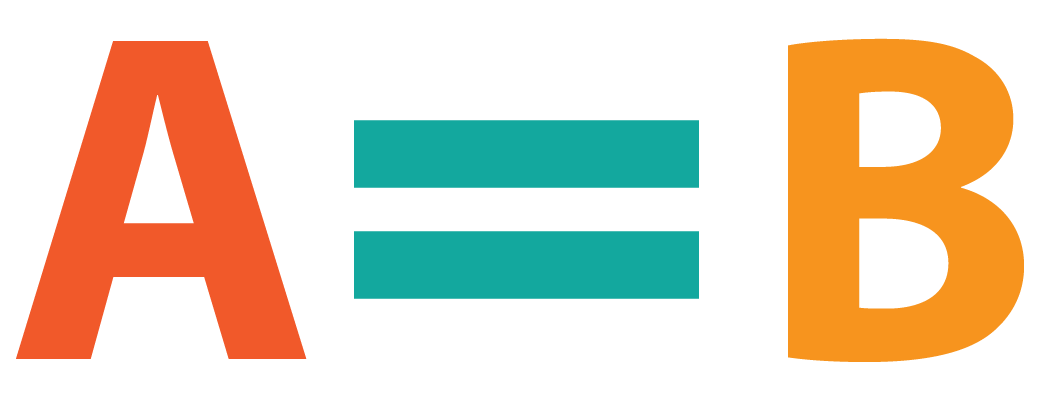# Equal Sign

Equal Sign

There are three different types of equal signs. Exactly equal is the one we’re all familiar with, but did you know there is a vertical equal sign, as well an approximately equal symbol. If you didn’t know yet, there is also less than or equal to symbol.

In this chapter, we will introduce you to each of these symbols and show you where they are used.

Check out the interactive simulations to know more about the lesson and try your hand at solving a few interesting practice questions at the end of the page.

## Lesson Plan

 1 What is Equal Sign? 2 Important Notes on Equal Sign 3 Solved Examples on Equal Sign 4 Challenging Questions on Equal Sign 5 Interactive Questions on Equal Sign

## What is Equal Sign?

The Equal sign is a sign of "Equality." Definition says that it is a mathematical symbol that is used to show equality in a distinct sense. So mathematically speaking, whenever we try to show equality between two expressions, we tend to "equate" the two items. Hence, the equal sign is the sign that helps us to mathematically show equality between two symbols.

## What is the Symbol of Equal to Sign

The symbol used to show the equal to sign is $$=$$ (two parallel horizontal lines).This symbol is only used when the two quantities to be compared are exactly the same. There are different symbols for each comparison aspect.

Click on the comparison aspects given here to know the symbols that are used.

Here is a list of some of the most commonly used comparison terms and their meanings:

Comparison Meaning
Equal to  Exactly the same
Not equal to Not exactly the same
Approximation Almost the same
Congruent Exactly the same appearance (shapes)
Similarity Same shapes, not exactly the same
Greater than equal to Greater than OR equal to

## Use of Equal to Sign in An Equation

The symbol of equal to is used to show the exact same amount of any two different quantities.For example, if we know that the price of 1 pound of meat is exactly \$3, we can say that

Price of 1 pound of meat $$=3$$

Keep in mind here that only exact values are to be given when using the equal to sign.

More Important Topics
Numbers
Algebra
Geometry
Measurement
Money
Data
Trigonometry
Calculus